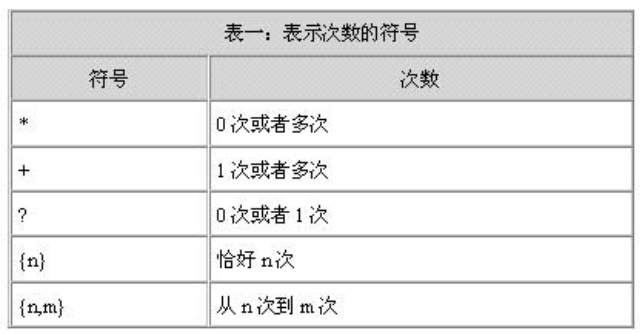# Java 正则表达式的用法和实例

## 一、概述：

• 用来描述或者匹配一系列符合某个语句规则的字符串

## 二、单个符号

1、英文句点.符号：匹配单个任意字符。

• 表达式t.o 可以匹配：tnot#oteo等等。不可以匹配：tnnotoTnot正o等。

2、中括号[]：只有方括号里面指定的字符才参与匹配，也只能匹配单个字符。

• 表达式：t[abcd]n 只可以匹配：tantbntcntdn。不可以匹配：thntabntn等。

3、| 符号。相当与“或”，可以匹配指定的字符，但是也只能选择其中一项进行匹配。

• 表达式：t(a|b|c|dd)n 只可以匹配：tantbntcntddn。不可以匹配taantntabcn等。

4、表示匹配次数的符号• 表达式：[0—9]{ 3 } \— [0-9]{ 2 } \— [0-9]{ 3 } 的匹配格式为：999—99—999
因为—符号在正则表达式中有特殊的含义，它表示一个范围，所以在前面加转义字符\

5、^符号：表示否，如果用在方括号内，^表示不想匹配的字符。

• 表达式：[^x] 第一个字符不能是x

6、\S符号：非空字符
7、\s符号：空字符，只可以匹配一个空格、制表符、回车符、换页符，不可以匹配自己输入的多个空格。
8、\r符号：空格符，与\n\tab相同

## 三、快捷符号

1、\d表示[0—9]
2、\D表示[^0—9]
3、\w表示[0—9A—Z_a—z]
4、\W表示[^0—9A—Z_a—z]
5、\s表示[\t\n\r\f]
6、\S表示[^\t\n\r\f]

## 四、常用的正则表达式

1、Java：(([a-z]|_)(\\w*)){6,20}匹配以字母或下划线开头，字母数字下划线结尾的字符串
2、JavaScript：/^(\-?)(\d+)$/匹配数字 3、JavaScript：/^\w+$/匹配字母数字下划线。

## 五、Java 中正则表达式的应用

1、判断功能

• public boolean matches(String regex)

package Lemon;

import java.util.Scanner;

public class RegexDm {
public static void main(String[] args){
Scanner sc = new Scanner(System.in);
System.out.println("请输入手机好：");
String s = sc.nextLine();

String regex = "1\\d{9}";//定义手机好规则
boolean flag = s.matches(regex);//判断功能
System.out.println("flag:"+flag);
}
}


2、分割功能

• public String[] split(String regex)

package Lemon;

import java.util.Scanner;

public class RegexDm {
public static void main(String[] args){
String age = "18-24";//定义年龄范围
String regex = "-";
String[] strArr = age.split(regex);//分割成字符串数组

int startAge = Integer.parseInt(strArr);
int endAge = Integer.parseInt(strArr);

Scanner sc = new Scanner(System.in);
System.out.println("请输入您的年龄：");
int a = sc.nextInt();
if (a >= startAge && a <= endAge){
System.out.println("你就是我想找的");
}else{
System.out.println("滚");
}
}
}


3、替换功能

• public String replaceAll(String regex,String replacement)

package Lemon;

public class RegexDm {
public static void main(String[] args){
String s = "12342jasfkgnas234";
//把字符串里面的数字替换成*
String regex = "\\d";
String ss = "*";
String result = s.replaceAll(regex,ss);
System.out.println(result);
}
}


## 六、常用正则表达式

• ^\d+$ ：非负整数（正整数 + 0） • ^[0-9]*[1-9][0-9]*$ ：正整数

• ^((-\d+)|(0+))$ ：非正整数（负整数 + 0） • ^-[0-9]*[1-9][0-9]*$ ：负整数

• ^-?\d+$ ：整数 • ^\d+(\.\d+)?$ ：非负浮点数（正浮点数 + 0）

• ^(([0-9]+\.[0-9]*[1-9][0-9]*)|([0-9]*[1-9][0-9]*\.[0-9]+)|([0-9]*[1-9][0-9]*))$ ：正浮点数 • ^((-\d+(\.\d+)?)|(0+(\.0+)?))$ ：非正浮点数（负浮点数 + 0）

• ^(-(([0-9]+\.[0-9]*[1-9][0-9]*)|([0-9]*[1-9][0-9]*\.[0-9]+)|([0-9]*[1-9][0-9]*)))$ ：负浮点数 • ^(-?\d+)(\.\d+)?$ ：浮点数

• ^[A-Za-z]+$ ：由26个英文字母组成的字符串 • ^[A-Z]+$ ：由26个英文字母的大写组成的字符串

• ^[a-z]+$ ：由26个英文字母的小写组成的字符串 • ^[A-Za-z0-9]+$ ：由数字和26个英文字母组成的字符串

• ^\w+$ ：由数字、26个英文字母或者下划线组成的字符串 • ^[\w-]+(\.[\w-]+)*@[\w-]+(\.[\w-]+)+$ ：email地址

• ^[a-zA-z]+://(\w+(-\w+)*)(\.(\w+(-\w+)*))*(\?\S*)?$ ：url • /^(d{2}|d{4})-((0([1-9]{1}))|(1[1|2]))-(([0-2]([1-9]{1}))|(3[0|1]))$/ ：年-月-日

• /^((0([1-9]{1}))|(1[1|2]))/(([0-2]([1-9]{1}))|(3[0|1]))/(d{2}|d{4})$/ ：月/日/年 • ^([w-.]+)@(([[0-9]{1,3}.[0-9]{1,3}.[0-9]{1,3}.)|(([w-]+.)+))([a-zA-Z]{2,4}|[0-9]{1,3})(]?)$ ：Emil

• /^((\+?[0-9]{2,4}\-[0-9]{3,4}\-)|([0-9]{3,4}\-))?([0-9]{7,8})(\-[0-9]+)?$/ ：电话号码 • ^(d{1,2}|1dd|2[0-4]d|25[0-5]).(d{1,2}|1dd|2[0-4]d|25[0-5]).(d{1,2}|1dd|2[0-4]d|25[0-5]).(d{1,2}|1dd|2[0-4]d|25[0-5])$ ：IP地址

• 匹配中文字符的正则表达式： [\u4e00-\u9fa5]

• 匹配双字节字符(包括汉字在内)：[^\x00-\xff]

• 匹配空行的正则表达式：\n[\s| ]*\r

• 匹配HTML标记的正则表达式：/<(.*)>.*<\/\1>|<(.*) \/>/

• 匹配首尾空格的正则表达式：(^\s*)|(\s*$) • 匹配Email地址的正则表达式：\w+([-+.]\w+)*@\w+([-.]\w+)*\.\w+([-.]\w+)* • 匹配网址URL的正则表达式：^[a-zA-z]+://(\\w+(-\\w+)*)(\\.(\\w+(-\\w+)*))*(\\?\\S*)?$

• 匹配帐号是否合法(字母开头，允许5-16字节，允许字母数字下划线)：^[a-zA-Z][a-zA-Z0-9_]{4,15}$ • 匹配国内电话号码：(\d{3}-|\d{4}-)?(\d{8}|\d{7})? • 匹配腾讯QQ号：^[1-9]*[1-9][0-9]*$

#### 元字符及其在正则表达式上下文中的行为：

• \ 将下一个字符标记为一个特殊字符、或一个原义字符、或一个后向引用、或一个八进制转义符。
• ^ 匹配输入字符串的开始位置。如果设置了 RegExp 对象的Multiline 属性，^ 也匹配 \n\r之后的位置。
• $ 匹配输入字符串的结束位置。如果设置了 RegExp 对象的Multiline 属性，$ 也匹配 \n\r之前的位置。
• * 匹配前面的子表达式零次或多次。
• + 匹配前面的子表达式一次或多次。+ 等价于 {1,}
• ? 匹配前面的子表达式零次或一次。? 等价于 {0,1}
• {n} n 是一个非负整数，匹配确定的n 次。
• {n,} n 是一个非负整数，至少匹配n 次。
• {n,m} m 和 n 均为非负整数，其中n <= m。最少匹配 n 次且最多匹配 m 次。在逗号和两个数之间不能有空格。
• ? 当该字符紧跟在任何一个其他限制符 (*, +, ?, {n}, {n,}, {n,m}) 后面时，匹配模式是非贪婪的。非贪婪模式尽可能少的匹配所搜索的字符串，而默认的贪婪模式则尽可能多的匹配所搜索的字符串。
• . 匹配除 \n之外的任何单个字符。要匹配包括 \n 在内的任何字符，请使用象 [.\n]的模式。
• (pattern) 匹配pattern 并获取这一匹配。
• (?:pattern) 匹配pattern 但不获取匹配结果，也就是说这是一个非获取匹配，不进行存储供以后使用。
• (?=pattern) 正向预查，在任何匹配 pattern 的字符串开始处匹配查找字符串。这是一个非获取匹配，也就是说，该匹配不需要获取供以后使用。
• (?!pattern) 负向预查，与(?=pattern)作用相反
• x|y 匹配 xy
• [xyz] 字符集合。
• [^xyz] 负值字符集合。
• [a-z] 字符范围，匹配指定范围内的任意字符。
• [^a-z] 负值字符范围，匹配任何不在指定范围内的任意字符。
• \b 匹配一个单词边界，也就是指单词和空格间的位置。
• \B 匹配非单词边界。
• \cx 匹配由x指明的控制字符。
• \d 匹配一个数字字符。等价于 [0-9]
• \D 匹配一个非数字字符。等价于 [^0-9]
• \f 匹配一个换页符。等价于 \x0c\cL
• \n 匹配一个换行符。等价于 \x0a\cJ
• \r 匹配一个回车符。等价于 \x0d\cM
• \s 匹配任何空白字符，包括空格、制表符、换页符等等。等价于[ \f\n\r\t\v]
• \S 匹配任何非空白字符。等价于 [^ \f\n\r\t\v]
• \t 匹配一个制表符。等价于 \x09\cI
• \v 匹配一个垂直制表符。等价于 \x0b\cK
• \w 匹配包括下划线的任何单词字符。等价于[A-Za-z0-9_]
• \W 匹配任何非单词字符。等价于 [^A-Za-z0-9_]
• \xn 匹配 n，其中 n 为十六进制转义值。十六进制转义值必须为确定的两个数字长。
• \num 匹配 num，其中num是一个正整数。对所获取的匹配的引用。
• \n 标识一个八进制转义值或一个后向引用。如果 \n 之前至少 n 个获取的子表达式，则 n 为后向引用。否则，如果 n 为八进制数字 (0-7)，则 n 为一个八进制转义值
• \nm 标识一个八进制转义值或一个后向引用。如果 \nm 之前至少有is preceded by at least nm 个获取得子表达式，则 nm 为后向引用。如果 \nm 之前至少有 n 个获取，则 n 为一个后跟文字 m 的后向引用。如果前面的条件都不满足，若 n 和 m 均为八进制数字 (0-7)，则 \nm 将匹配八进制转义值 nm
• \nml 如果 n 为八进制数字 (0-3)，且 ml 均为八进制数字 (0-7)，则匹配八进制转义值 nml
• \un 匹配 n，其中 n 是一个用四个十六进制数字表示的Unicode字符。
• 匹配中文字符的正则表达式： [u4e00-u9fa5]
• 匹配双字节字符(包括汉字在内)：[^x00-xff]
• 匹配空行的正则表达式：n[s| ]*r
• 匹配HTML标记的正则表达式：/<(.*)>.*</1>|<(.*) />/
• 匹配首尾空格的正则表达式：(^s*)|(s*$) • 匹配Email地址的正则表达式：w+([-+.]w+)*@w+([-.]w+)*.w+([-.]w+)* • 匹配网址URL的正则表达式：http://([w-]+.)+[w-]+(/[w- ./?%&=]*)? #### 利用正则表达式限制网页表单里的文本框输入内容： • 用正则表达式限制只能输入中文：onkeyup="value=value.replace(/[^u4E00-u9FA5]/g,'')" onbeforepaste="clipboardData.setData('text',clipboardData.getData('text').replace(/[^u4E00-u9FA5]/g,''))" • 用正则表达式限制只能输入全角字符： onkeyup="value=value.replace(/[^uFF00-uFFFF]/g,'')" onbeforepaste="clipboardData.setData('text',clipboardData.getData('text').replace(/[^uFF00-uFFFF]/g,''))" • 用正则表达式限制只能输入数字：onkeyup="value=value.replace(/[^d]/g,'') "onbeforepaste="clipboardData.setData('text',clipboardData.getData('text').replace(/[^d]/g,''))" • 用正则表达式限制只能输入数字和英文：onkeyup="value=value.replace(/[W]/g,'') "onbeforepaste="clipboardData.setData('text',clipboardData.getData('text').replace(/[^d]/g,''))" #### 整理： • 匹配中文字符的正则表达式： [\u4e00-\u9fa5] • 匹配双字节字符(包括汉字在内)：[^\x00-\xff] • 匹配空行的正则表达式：\n[\s| ]*\r • 匹配HTML标记的正则表达式：/<(.*)>.*<\/\1>|<(.*) \/>/ • 匹配首尾空格的正则表达式：(^\s*)|(\s*$)

• 匹配IP地址的正则表达式：/(\d+)\.(\d+)\.(\d+)\.(\d+)/g //

• 匹配Email地址的正则表达式：\w+([-+.]\w+)*@\w+([-.]\w+)*\.\w+([-.]\w+)*

• 匹配网址URL的正则表达式：http://(/[\w-]+\.)+[\w-]+(/[\w- ./?%&=]*)?

• sql语句：^(select|drop|delete|create|update|insert).*$ • 非负整数：^\d+$

• 正整数：^[0-9]*[1-9][0-9]*$ • 非正整数：^((-\d+)|(0+))$

• 负整数：^-[0-9]*[1-9][0-9]*$ • 整数：^-?\d+$

• 非负浮点数：^\d+(\.\d+)?$ • 正浮点数：^((0-9)+\.[0-9]*[1-9][0-9]*)|([0-9]*[1-9][0-9]*\.[0-9]+)|([0-9]*[1-9][0-9]*))$

• 非正浮点数：^((-\d+\.\d+)?)|(0+(\.0+)?))$ • 负浮点数：^(-((正浮点数正则式)))$

• 英文字符串：^[A-Za-z]+$ • 英文大写串：^[A-Z]+$

• 英文小写串：^[a-z]+$ • 英文字符数字串：^[A-Za-z0-9]+$

• 英数字加下划线串：^\w+$ • E-mail地址：^[\w-]+(\.[\w-]+)*@[\w-]+(\.[\w-]+)+$

• URL：^[a-zA-Z]+://(\w+(-\w+)*)(\.(\w+(-\w+)*))*(\?\s*)?$ 或者：^http:\/\/[A-Za-z0-9]+\.[A-Za-z0-9]+[\/=\?%\-&_~@[\]\':+!]*([^<>\"\"])*$

• 邮政编码：^[1-9]\d{5}$ • 中文：^[\u0391-\uFFE5]+$

• 电话号码：^(($$\d{2,3}$$)|(\d{3}\-))?($$0\d{2,3}$$|0\d{2,3}-)?[1-9]\d{6,7}(\-\d{1,4})?$ • 手机号码：^(($$\d{2,3}$$)|(\d{3}\-))?13\d{9}$

• 双字节字符(包括汉字在内)：^\x00-\xff

• 匹配首尾空格：(^\s*)|(\s*$)（像vbscript那样的trim函数） • 匹配HTML标记：<(.*)>.*<\/\1>|<(.*) \/> • 匹配空行：\n[\s| ]*\r • 提取信息中的网络链接：(h|H)(r|R)(e|E)(f|F) *= *('|")?(\w|\\|\/|\.)+('|"| *|>)? • 提取信息中的邮件地址：\w+([-+.]\w+)*@\w+([-.]\w+)*\.\w+([-.]\w+)* • 提取信息中的图片链接：(s|S)(r|R)(c|C) *= *('|")?(\w|\\|\/|\.)+('|"| *|>)? • 提取信息中的IP地址：(\d+)\.(\d+)\.(\d+)\.(\d+) • 提取信息中的中国手机号码：(86)*0*13\d{9} • 提取信息中的中国固定电话号码：($$\d{3,4}$$|\d{3,4}-|\s)?\d{8} • 提取信息中的中国电话号码（包括移动和固定电话）：($$\d{3,4}$$|\d{3,4}-|\s)?\d{7,14} • 提取信息中的中国邮政编码：[1-9]{1}(\d+){5} • 提取信息中的浮点数（即小数）：(-?\d*)\.?\d+ • 提取信息中的任何数字 ：(-?\d*)(\.\d+)? • IP地址：(\d+)\.(\d+)\.(\d+)\.(\d+) • 电话区号：/^0\d{2,3}$/

• 腾讯QQ号：^[1-9]*[1-9][0-9]*$ • 帐号(字母开头，允许5-16字节，允许字母数字下划线)：^[a-zA-Z][a-zA-Z0-9_]{4,15}$

• 中文、英文、数字及下划线：^[\u4e00-\u9fa5_a-zA-Z0-9]+\$

## 七、实例

1、效验QQ号（要求：5-15位数字，0不能开头）

package Lemon;

import java.util.Scanner;

public class RegexDm {
public static void main(String[] args){
Scanner sc = new Scanner(System.in);
System.out.println("请输入您的QQ号码：");
String qq = sc.nextLine();
System.out.println("checkQQ:"+checkQQ(qq));
}

private static boolean checkQQ(String qq) {
return qq.matches("[1-9]{1}\\d{4,14}");
}
}06-058
06-056
06-0511
06-0533
05-1842
05-1236
05-1244
04-0334
04-0332
11-10214
11-07980
07-231143
07-19249
07-10249
07-10195
07-09965
06-28174
06-19374
06-19216
06-03233